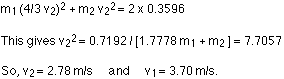## Friday, 3 October 2014

### Two positively-charged balls - FINDING VELOCITY CONSERVATION OF ENERGY

Two positively-charged balls are tied together by a string. One ball has a mass of 30 g and a charge of 1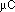; the other has a mass of 40 g and a charge of 2. The distance between them is 5 cm. The ball with the smaller charge has a mass of 30 g; the other ball has a mass of 40 g. Initially they are at rest, but when the string is cut they move apart. When they are a long way away from each other, how fast are they going?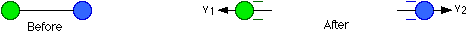Let's start by looking at energy. No external forces act on this system of two charges, so the energy must be conserved. To start with all the energy is potential energy; this will be converted into kinetic energy.
Energy at the start : KE = 0
PE = k q Q / r = (8.99 x 109) (1 x 10-6) (2 x 10-6) / 0.05 = 0.3596 J
When the balls are very far apart, the r in the equation for potential energy will be large, making the potential energy negligibly small.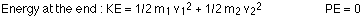Energy is conserved, so the kinetic energy at the end is equal to the potential energy at the start: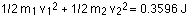The masses are known, but the two velocities are not. To solve for the velocities, we need another relationship between them. Because no external forces act on the system, momentum will also be conserved. Before the string is cut, the momentum is zero, so the momentum has to be zero all the way along. The momentum of one ball must be equal and opposite to the momentum of the other, so: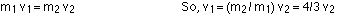Plugging this into the energy equation gives: